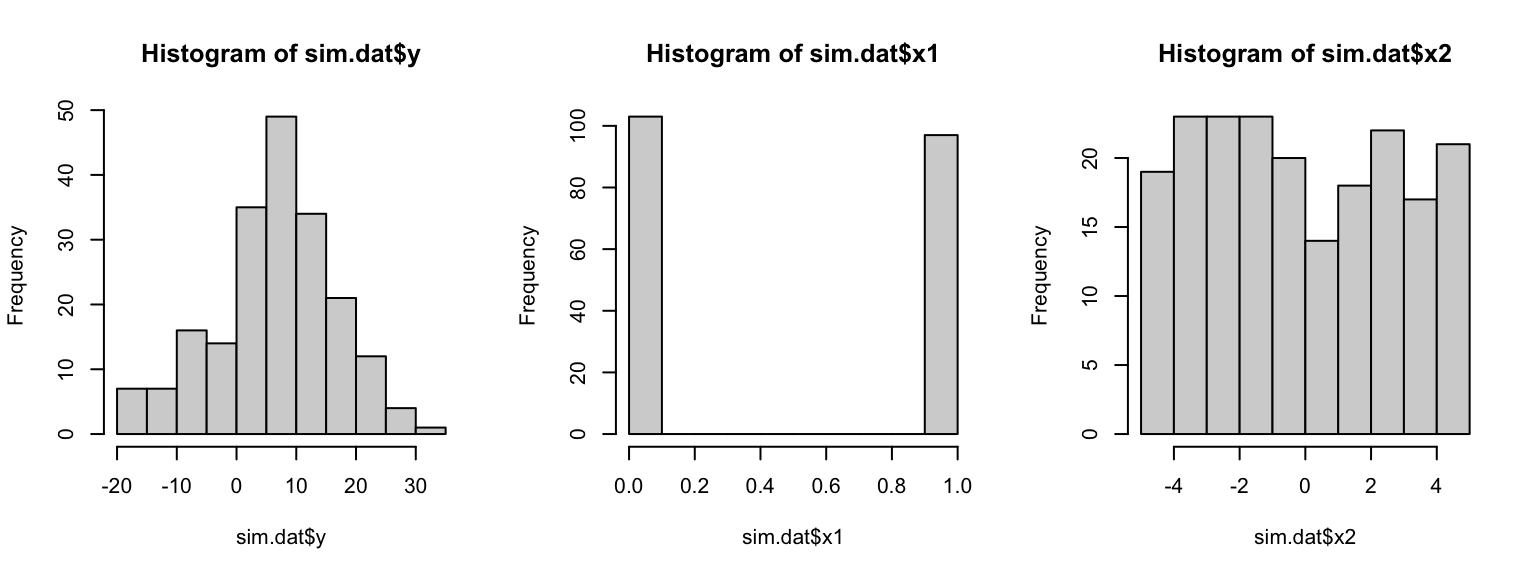This tutorial shows you:

• how to specify regression models with interaction terms
• (briefly) how to interpret interaction terms (refer to AR ch. 7 and Brambor et al. for a more detailed treatment)
• how to graph marginal/conditional effects from regression estimates

As always, please note that this tutorial only accompanies the other course materials and that you are expected to have worked through assigned reading before tackling this tutorial.

Note: You must have read Brambor, Clark & Golder (2006) before working on this tutorial.

# Interaction terms in regression

Interaction terms enter the regression equation as the product of two constitutive terms, $$x_1$$ and $$x_2$$. For this product term $$x_1 x_3$$, the regression equation adds a separate coefficient $$\beta_3$$.

$y = \alpha + \beta_1 x_1 + \beta_2 x_2 + \beta_3 x_1 x_2 + \varepsilon$

# Example 1: one binary, one continuous term

In thinking about interaction terms, it helps to first simplify by working through the prediction of the regression equation for different values of two predictors, $$x_1$$ and $$x_2$$. We can imagine a continuous outcome $$y$$, e.g. the income of Hollywood actors, that we predict with two variables. The first, $$x_1$$, is a binary variable such as female gender; it takes on values of 0 (males) and 1 (females) only. The second, $$x_2$$, is a continuous variable, ranging from $$-5$$ to $$+5$$, such as a (centered and standardized) measure of age. In this case, I’m using the term “effect” loosely and non-causally. An interaction term expresses the idea that the effect of one variable depends on the value of the other variable. With these variables, this suggests that effect of age on actors’ income is different for male actors than for female actors.

• $$\beta_1$$ is the effect of $$x_1$$ on $$y$$ when $$x_2$$ is 0:

• $$\hat{y} = \alpha + \beta_1 x_1 + \beta_2 \times 0 + \beta_3 x_1 \times 0$$
• $$\hat{y} = \alpha + \beta_1 x_1 + 0 + 0$$
• $$\beta_2$$ is the effect of $$x_2$$ on $$y$$ when $$x_1$$ is 0:

• $$\hat{y} = \alpha + \beta_1 \times 0 + \beta_2 x_2 + \beta_3 \times 0 \times x_2$$
• $$\hat{y} = \alpha + \beta_2 x_2 + 0$$
• When both $$x_1$$ and $$x_2$$ are not 0, $$\beta_3$$ becomes important, and the effect of $$x_1$$ now varies with the value of $$x_2$$. We can plug in 1 for $$x_1$$ and simplify the equation as follows:

• $$\hat{y} = \alpha + \beta_1 \times 1 + \beta_2 x_2 + \beta_3 \times 1 \times x_2$$
• $$\hat{y} = \alpha + \beta_1 + \beta_2 x_2 + \beta_3 \times x_2$$
• $$\hat{y} = (\alpha + \beta_1) + (\beta_2 + \beta_3) \times x_2$$

With simulated data, this can be illustrated easily. I begin by simulating a dataset with 200 observations, two predictors $$x_1$$ (binary: male/female) and $$x_2$$ (continuous: standardized and centered age), and create $$y$$ (continuous: income) as the linear combination of $$x_1$$, $$x_2$$, and an interaction term of the two predictors.

set.seed(123)
n.sample <- 200
x1 <- rbinom(n.sample, size = 1, prob = 0.5)
x2 <- runif(n.sample, -5, 5)
a <- 5
b1 <- 3
b2 <- 4
b3 <- -3
e <- rnorm(n.sample, 0, 5)
y <- a + b1 * x1 + b2 * x2 + b3 * x1 * x2 + e
sim.dat <- data.frame(y, x1, x2)

Before advancing, this is what the simulated data look like:

par(mfrow = c(1, 3))
hist(sim.dat$y) hist(sim.dat$x1)
hist(sim.dat\$x2)par(mfrow = c(1, 1))

For convenience, you could also use the multi.hist() function from the “psych” package. It automatically adds a density curve (dashed line) and a normal density plot (dotted line).

library("psych")
multi.hist(sim.dat, nrow = 1)